## Strong Pseudoprime

A strong pseudoprime to a baseis an Odd Composite Numberwith(forOdd) for which either(1)

or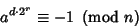(2)

for some.

The definition is motivated by the fact that a Fermat Pseudoprimeto the basesatisfies(3)

But sinceis Odd, it can be written, and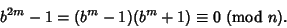(4)

Ifis Prime, it must Divide at least one of the Factors, but can't Divide both because it would then Divide their difference(5)

Therefore,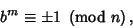(6)

so writeto obtain(7)

IfDivides exactly one of these Factors but is Composite, it is a strong pseudoprime. A Composite number is a strong pseudoprime to at most 1/4 of all bases less than itself (Monier 1980, Rabin 1980). The strong pseudoprimes provide the basis for Miller's Primality Test and Rabin-Miller Strong Pseudoprime Test.

A strong pseudoprime to the baseis also an Euler Pseudoprime to the base(Pomerance et al. 1980). The strong pseudoprimes include some Euler Pseudoprimes, Fermat Pseudoprimes, and Carmichael Numbers.

There are 4842 strong psp(2) less than, where a psp(2) is also known as a Poulet Number. The strong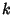-pseudoprime test for, 3, 5 correctly identifies all Primes belowwith only 13 exceptions, and if 7 is added, then the only exception less thanis 315031751. Jaeschke (1993) showed that there are only 101 strong pseudoprimes for the bases 2, 3, and 5 less than, nine if 7 is added, and none if 11 is added. Also, the bases 2, 13, 23, and 1662803 have no exceptions up to.

Ifis Composite, then there is a base for whichis not a strong pseudoprime. There are therefore no strong Carmichael Numbers.'' Letdenote the smallest strong pseudoprime to all of the firstPrimes taken as bases (i.e, the smallest Odd Number for which the Rabin-Miller Strong Pseudoprime Test on bases less than or equal tofails). Jaeschke (1993) computedfromto 8 and gave upper bounds forto 11.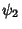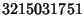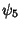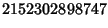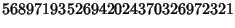(Sloane's A014233). A seven-step test utilizing these results (Riesel 1994) allows all numbers less thanto be tested.

Pomerance et al. (1980) have proposed a test based on a combination of Strong Pseudoprimes and Lucas Pseudoprimes. They offer a \$620 reward for discovery of a Composite Number which passes their test (Guy 1994, p. 28).

See also Carmichael Number, Miller's Primality Test, Poulet Number, Rabin-Miller Strong Pseudoprime Test, Rotkiewicz Theorem, Strong Elliptic Pseudoprime, Strong Lucas Pseudoprime

References

Baillie, R. and Wagstaff, S. Lucas Pseudoprimes.'' Math. Comput. 35, 1391-1417, 1980.

Guy, R. K. Pseudoprimes. Euler Pseudoprimes. Strong Pseudoprimes.'' §A12 in Unsolved Problems in Number Theory, 2nd ed. New York: Springer-Verlag, pp. 27-30, 1994.

Jaeschke, G. On Strong Pseudoprimes to Several Bases.'' Math. Comput. 61, 915-926, 1993.

Monier, L. Evaluation and Comparison of Two Efficient Probabilistic Primality Testing Algorithms.'' Theor. Comput. Sci. 12, 97-108, 1980.

Pomerance, C.; Selfridge, J. L.; and Wagstaff, S. S. Jr. The Pseudoprimes to.'' Math. Comput. 35, 1003-1026, 1980. Available electronically from ftp://sable.ox.ac.uk/pub/math/primes/ps2.Z.

Rabin, M. O. Probabilistic Algorithm for Testing Primality.'' J. Number Th. 12, 128-138, 1980.

Riesel, H. Prime Numbers and Computer Methods for Factorization, 2nd ed. Basel: Birkhäuser, p. 92, 1994.

Sloane, N. J. A. Sequence A014233 in The On-Line Version of the Encyclopedia of Integer Sequences.'' http://www.research.att.com/~njas/sequences/eisonline.html.